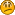###Author Topic: What hapenning with my C::B  (Read 1195 times)

#### Yeyasu

• Guest##### What hapenning with my C::B
« on: April 03, 2019, 02:21:01 am »
Hello, I have a "problem" when I was programing with C::B, I going to try to express me well because my mother language is Spanish.

Well, I have this code:

Code
``#include <iostream>using namespace std;int main(int argc, char** argv) {	cout << "Inserte los datos de la matriz" << endl;	int m;	for (int i=0; i<4; i++) {		for(int j=0; j<3; j++) {			cin >> m[i][j];		}	}	for (int i=0; i<4; i++) {		for(int j=0; j<3; j++) {			cout << m[i][j];		}		cout << endl;	}	int s;	int con=0;	for (int i=0; i<4; i++) {		for(int j=0; j<3; j++) {			con= con+m[i][j];		}		s[i]=con;		con=0;	}	int mayor=0;	for (int i=0; i<4; i++) {		if (mayor<s[i]) {			mayor=s[i];		}	}	cout << "La fila con mayor sumatoria es " << mayor<< endl;	int fm=0;	for (int i=0; i<4; i++) {		for(int j=0; j<3; j++) {			fm= fm+m[i][j];		}		if(fm==mayor) {			for(int b=0; b<3; b++) {				cout << m[i][b];			}		}		fm=0;	}return 0;}``

Basically shows the row where the sum of its components is higher, for example:

1 1 1
2 2 2
3 3 3
4 4 4
The row with the highest sum is 12 (4+4+4)
and then shows the row
4 4 4

Now the "problem"
C::B shows me thisAnd Dev-C++ shows thisCan you see that "657" in the output of C::B?
I broke my head for hours trying to find the error in my logic, but the logic is fine, so a run my code in Dev-C++, and it's ok

What is happen with C::B?? I don't understand

I want to use C::B, but this "problem" make me lost a lot of time, I afraid that this happen again whith other code####sodev

• Regular
•• Posts: 424##### Re: What hapenning with my C::B
« Reply #1 on: April 03, 2019, 05:39:23 am »
Nothing is happening with your C::B, it is working fine i guess? It can run the selected compiler, can execute the created program, all good. The same applies to Dev-C++ i assume. Or do these pictures i cant see show any errors like cant launch compiler, or any error output of the compiler?

CodeBlocks is an IDE (without an integrated compiler, not sure about that other IDE you are using), so unless you cant write your code, cant execute the compiler, this is not a CodeBlocks error and off-topic for this forum!

Your code is broken! Apparently you dont use the same compiler in both IDEs or not the same settings (i guess debug vs. release) so the buffer overrun on s is causing a different defect!